## Example Questions

### Example Question #1 : How To Find A Ratio Of Square Roots

x= 100

If x is placed on a number line, what two integers is it between?

2 and 3

5 and 6

3 and 4

Cannot be determined

4 and 5

3 and 4

Explanation:

It might be a little difficult taking a fourth root of 100 to isolate x by itself; it might be easier to select an integer and take that number to the fourth power. For example 3= 81 and 4= 256. Since 34 is less than 100 and 44 is greater than 100, x would lie between 3 and 4.

### Example Question #2 : How To Find A Ratio Of Square Roots

What is the ratio of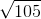to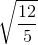?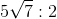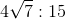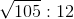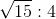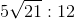Explanation:

The ratio of two numbers is merely the division of the two values. Therefore, for the information given, we know that the ratio oftocan be rewritten: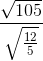Now, we know that the square root in the denominator can be "distributed" to the numerator and denominator of that fraction: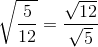Thus, we have: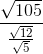To divide fractions, you multiply by the reciprocal: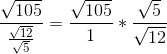Now, since there is onein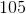, you can rewrite the numerator: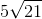This gives you: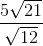Rationalize the denominator by multiplying both numerator and denominator by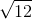: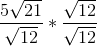Let's be careful how we write the numerator so as to make explicit the shared factors: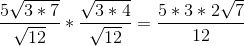Now, reduce: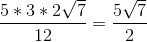This is the same as### Example Question #3 : How To Find A Ratio Of Square Roots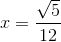and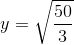What is the ratio ofto?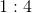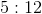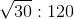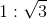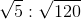Explanation:

To find a ratio like this, you need to divideby. Recall that when you have the square root of a fraction, you can "distribute" the square root to the numerator and the denominator. This lets you rewriteas: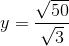Next, you can write the ratio of the two variables as: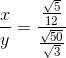Now, when you divide by a fraction, you can rewrite it as the multiplication by the reciprocal. This gives you: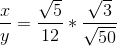Simplifying, you get: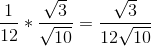You should rationalize the denominator: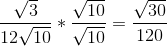This is the same as: